# Schreiber Higher Structures

Contents

Higher Structures in Mathematics and Physics

an introductory talk held at:

1. Meeting of Maths@CAS Brno, 2016 Nov 9-11

2. Oberwolfach Workshop 1651a, 2016 Dec. 18-23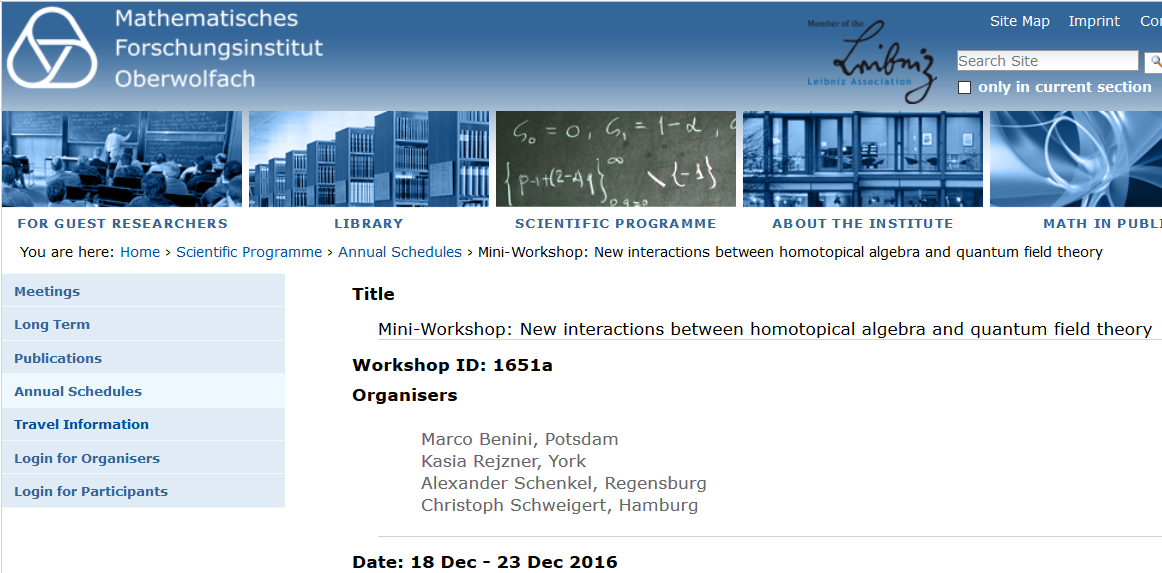# Contents

## Higher structures

$\,$

The term “higher structures” is short for

mathematical structures on higher homotopy types.

$\,$

We first explain what this means.

$\,$

Abstract homotopy

Consider a set

$X$

Given two elements

$x,y \in X$

there is the proposition

$x = y$

Either $x$ is equal to $y$, or it is not.

But there may be more than one

• way how they are equal,

• i.e. reason that they are equal,

• i.e. proof that they are equal.

Let’s write

$x \stackrel{\gamma}{\longrightarrow} y$

for one such way.

Call this a homotopy from $x$ to $y$.

Let’s write

$Id_X(x,y)$

for the set of homotopies from $x$ to $y$ in $X$.

Now the same reasoning applies to homotopies: given

$\gamma_1, \gamma_2 \in Id_X(x,y)$

two homotopies, there is the proposition

$\gamma_1 = \gamma_2$

Either they are equal or not. But again there may be more than one way/reason/proof $\kappa$ that exhibits their equality:

$\gamma_1 \stackrel{\kappa}{\longrightarrow} \gamma_2 \,.$

This may be called a higher homotopy.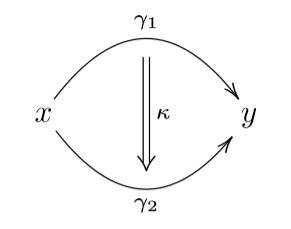And it keeps going this way: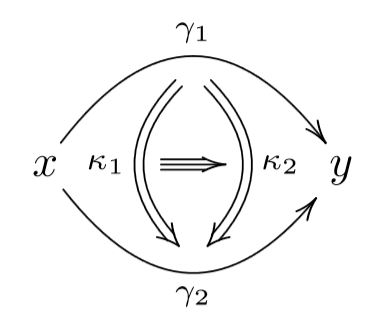And so on.

$\,$

Taking into account all these higher homotopies, the original “set” $X$ is in general richer than a set in the sense of ZFC.

To avoid clash of terminology, $X$ is called a type, or homotopy type.

$\,$

One formalization of this picture is due to

This is mostly known as the theory of “model categories”,

short for “categories of models for homotopy types”.

$\,$

Another formalization of this picture is Martin-Löf type theory (Martin-Löf 73), a kind of intuitionistic constructive set theory.

That this is secretly a formalization of abstract homotopy theory was only understood more recently (Hofmann-Streicher 98, Awodey-Warren 07, Kapulkin-Lumsdaine 12)

Ever since it is also called homotopy type theory (UFP 13).

$\,$

Topological homotopy

The classical model that motivated abstract homotopy theory is the homotopy theory of topological spaces.

Given two continuous functions from a topological space $X$ space to a topological space $Y$

$f,g \;\colon\; X \longrightarrow Y$

hence given two points in the mapping space

$f,g \in Maps(X,Y)$

then a homotopy $\eta$ between them

$f \stackrel{\eta}{\longrightarrow} g$is a continuous family of continuous paths between the points

$\array{ X \times \{0\} \\ \downarrow & \searrow^{\mathrlap{f}} \\ X \times [0,1] &\overset{\eta}{\longrightarrow}& Y \\ \uparrow & \nearrow_{\mathrlap{g}} \\ X \times \{1\} }$

(graphics grabbed from J. Tauber here)

In this perspective topological spaces are called homotopy types.

A homotopy n-type is a space inside which there exist higher homotopies that are non-trivial up to higher homotopy only up to order $n$.

$\,$

Hence an ordinary set is equivalently a homotopy 0-type.

The rest is “higher homotopy types”.

$\,$

Now a “higher structure” is a structure on higher homotopy types:

$\,$

Higher structures

$\,$

A mathematical structure, à la Bourbaki, is

1. a collection of sets

2. equipped with functions between them,

3. subject to equations between these functions.

$\,$

Example. A group is

1. a set $G$

2. equipped with

1. multiplication: a function $\;\;(-)\cdot(-) \colon G \times G \to G\;\;$

2. neutral element: a function $\;\;e \colon \ast \to G\;\;$

3. inverses: a function $\;\;(-)^{-1} \colon G \to G\;\;$

3. such that this satisfies the equations for

1. associativity: $(x \cdot y) \cdot z = x \cdot (y \cdot z)$

2. unitality: $x \cdot e = e \cdot x = x$

3. invertibility: $x^{-1} \cdot x = e$.

$\,$

A higher structure is like a Bourbakian mathematical structure but

Here “coherence” is the condition that “iterated structure homotopies are unique up to homotopy”.

$\,$

Example. A “first step higher group”, called a 2-group (Sinh 73, Baez-Lauda 03), is a group structure on a homotopy 1-type.

So the associativity equality is to be replaced by a choice of homotopy

$(x \cdot y) \cdot z \stackrel{a_{x,y,z}}{\longrightarrow} x \cdot (y \cdot z)$

called the associator.

The coherence to be imposed on this is the condition that the composite homotopy

$((w \cdot x) \cdot y) \cdot z \longrightarrow w \cdot (x \cdot (y \cdot z))$

is unique, in that the following two ways of composing associators to achieves this are related by a homotopy-of-homotopies, hence an equality (by assumption that we have just a 1-type).

This coherence condition is called the pentagon identity.

It implies coherence in the following sense:

$\,$

Coherence theorem for 2-groups (MacLane 63): All homotopies of re-bracketing any expression, using the given associators, coincide.

$\,$

Example (delooping 2-group)

For $G$ a discrete group, there is its classifying space denoted

$B G$

or

$K(G,1)$

For $X$ a (paracompact) topological space, then homotopy classes of continuous functions into $B G$ classify $G$-principal covering spaces of $X$:

$\left\{X \longrightarrow B G \right\} \;\; \simeq \;\; \left\{ \; G\text{-principal coverings of} \; X \; \right\} \,.$

If $G$ happens to be an abelian group, then $B G$ is a 2-group. The multiplication

$B G \times B G \longrightarrow B G$

is the map which classifies the tensor product of $G$-coverings regarded as group ring-module bundles.

The existence of inverses reflects the fact that as such each $G$-covering corresponds to a line bundle.

$\,$

Higher geometric groups

Just like

so

and generally

(e.g. Schreiber 13)

$\,$

$\,,$

Higher principal bundles

All the usual constructions done with ordinary groups now have their higher homotopy theoretic analogs.

For instance a “parameterized group” – called a $G$-principal bundle – is a space

$P$

equipped with a $G$-action

$G \times P \longrightarrow P$

over some space $X$

$\array{ P \\ \downarrow \\ X }$

such that over some open cover $\{U_i \to X\}$ then there are $G$-equivariant equivalences

$P|_{U_i} \stackrel{\simeq}{\longrightarrow} U_i \times G$

over each chart $U_i$.

For example the frame bundle $Fr(X)$ of a smooth manifold $X$ of dimension $n$ is an general linear group-principal bundle

$\array{ GL(n) &\hookrightarrow& Fr(X) \\ && \downarrow \\ && X }$

The higher coherent homotopy version of this definition yields

the concept of principal infinity-bundles (Nikolaus-Schreiber-Stevenson 12).

For instance a principal 2-bundle over a delooping 2-group of the form $\mathbf{B}A$ as above

$\array{ \mathbf{B}A &\hookrightarrow& P \\ && \downarrow \\ && X }$

is what is often called a bundle gerbe with band $A$.

We will see examples of higher principal bundles naturally appear in the following.

$\,$

Higher categorical structures

All higher homotopies are invertible, up to homotopy.

For example the inverse-up-to-homotopy of a topological homotopy

$X \times [0,1] \stackrel{\eta}{\longrightarrow} Y$

is the “reversed” map

$X \times [0,1] \stackrel{(x,t) \mapsto (x,1-t)}{\longrightarrow} X \times [0,1] \stackrel{\eta}{\longrightarrow} Y \,.$

$\,$

But one may generalize further and consider non-invertible homotopies up to some order $\lt n$.

The result might be called “directed homotopy theory”.

But one speaks of higher categories, specifically (infinity,n)-categories.

This is more complicated than plain higher homotopy theory. The latter is the special case of (infinity,1)-categories.

More generally then:

$\,$

A higher structure is a mathematical structure internal to an (infinity,n)-category.

$\,$

This is also called categorification (Crane-Yetter 96, Baez-Dolan 98).

For a long time the relevant theory had been mostly missing, but now it exists (Lurie 1-).

A key application and motivation for this are extended topological quantum field theories.

$\,$

Here we do not further dwell on such “directed higher structures”.

$\,$

## dg-Structures

$\,$

Various “shadows” of higher homotopy theory are more widely familiar.

One example is chain complexes.

$\,$

Consider a chain complex in non-negative degree.

$\left[ \cdots \stackrel{\partial}{\to} V_n \stackrel{\partial}{\to} \cdots \stackrel{\partial}{\to} V_1 \stackrel{\partial}{\to} V_0 \right] \;\;\;\;\;\,,\;\;\; \partial \circ \partial = 0 \,.$

Let

$x,y \in V_0$

be two elements in degree 0, whose images in degree-0 chain homology

$[x], [y] \;\in H_0(V_\bullet) \coloneqq V_0/im(\partial|_{V_1})$

are equal

$[x] = [y] \,.$

This means that there exists an element

$\gamma \in V_1$

in degree 1 such that

$\partial \gamma = y - x$

(a coboundary).

Hence every such $\gamma$ is a reason for the equality, hence a homotopy

$\left( \; \partial \gamma = y - x \; \right) \;\;\Leftrightarrow\;\; \left( x \stackrel{\gamma}{\longrightarrow} y \right) \,.$

Next, let

$\gamma_1, \gamma_2 \in V_1$

be two coboundaries, both between $x$ and $y$. Then an element

$\kappa \in V_2$

with

$\partial \kappa = \gamma_2 - \gamma_1$

is a way for them to be equalNamely this is a way for them to be equal in degree-1 chain homology

$H_1(V_\bullet) \coloneqq ker(\partial|_{V_1})/im(\partial|_{V_2})$

in the sense that

$ = [\gamma_2 - \gamma_1]$

witnessed by

$0 \stackrel{\kappa}{\longrightarrow} (\gamma_2 -\gamma_1) \,.$

Next, an element in $V_3$ defines a third order homotopyand so on.

$\,$

This way each chain complex defines a homotopy type.

This is called the Dold-Kan correspondence (Dold 58, Kan 58).

$\,$

$\,$

Example (Poincaré lemma)

For $X$ a smooth manifold of dimension $n$, its de Rham complex is the chain complex

$\Omega^\bullet(X) \;\coloneqq\; \left[ C^\infty(X) = \Omega^0(X) \stackrel{d_{dR}}{\to} \Omega^1(X) \stackrel{d_{dR}}{\to} \cdots \stackrel{d_{dR}}{\to} \Omega^n(X) \right]$

of differential forms with the de Rham differential between them. If $X$ is an open ball, then there is a homotopy equivalence from the de Rham complex to

$\mathbb{R}[n] \;\coloneqq\; \left[ \underset{deg \, n}{\underbrace{ \mathbb{R} }} \stackrel{0}{\to} 0 \stackrel{0}{\to} \cdots \stackrel{0}{\to} 0 \right] \,.$

namely chain homotopies of the form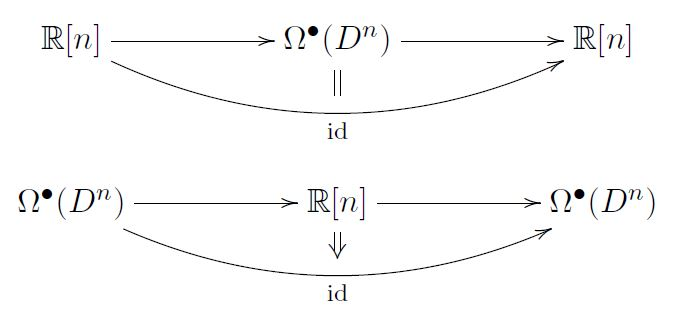This is the statement of the Poincaré lemma. The second chain homotopy is traditionally known as the homotopy operator used in the standard proof of the Poincaré lemma.

$\,$

$\,$

In conclusion, there are higher mathematical structures on chain complexes.

These are often called differential graded structures, or dg-structures for short.

$\,$

Here is a key example:

$\,$

Higher Lie algebras

$\,$

Recall the mathematical structure called a Lie algebra:

Example A Lie algebra is

1. a vector space $V$

2. equipped with a function

• Lie bracket: $[-,-] \;\colon\; V \times V \longrightarrow V$
3. such that

1. skew-symmetry: $[x,y] = - [y,x]$

2. bilinearity: $[k_1 v_1 + k_2 v_2, w] = k_1 [v_1, w] + k_2 [v_2,w]$

3. Jacobi identity: $[x,[y,z]] = [[x,y], z] + [y,[x,z]]$.

$\,$

A higher homotopy Lie algebra structure in the homotopy theory of chain complexes, is called equivalently a

$\,$

Such a higher Lie algebra consists, first of all, of a skew-symmetric chain map

$[-,-] \;\colon\; V \otimes V \longrightarrow V$

$[x,y] = -(-1)^{deg(x)deg(y)}\, [y,x]$

bilinear map that respects the differential

$[\partial x, y] + (-1)^{deg(x)} [x, \partial y] = \partial[x,y]$

and which satisfies the Jacobi identity up to a specified homotopy called the Jacobiator

$[x,[y,z]] \;\;\overset{j_{x,y,z}}{\longrightarrow}\;\; [[x,y],z] + (-1)^{deg(x)deg(y)} [y,[x,z]] \,.$

Then there is a coherence condition which says that the two possible ways of re-bracketing four elements are homotopic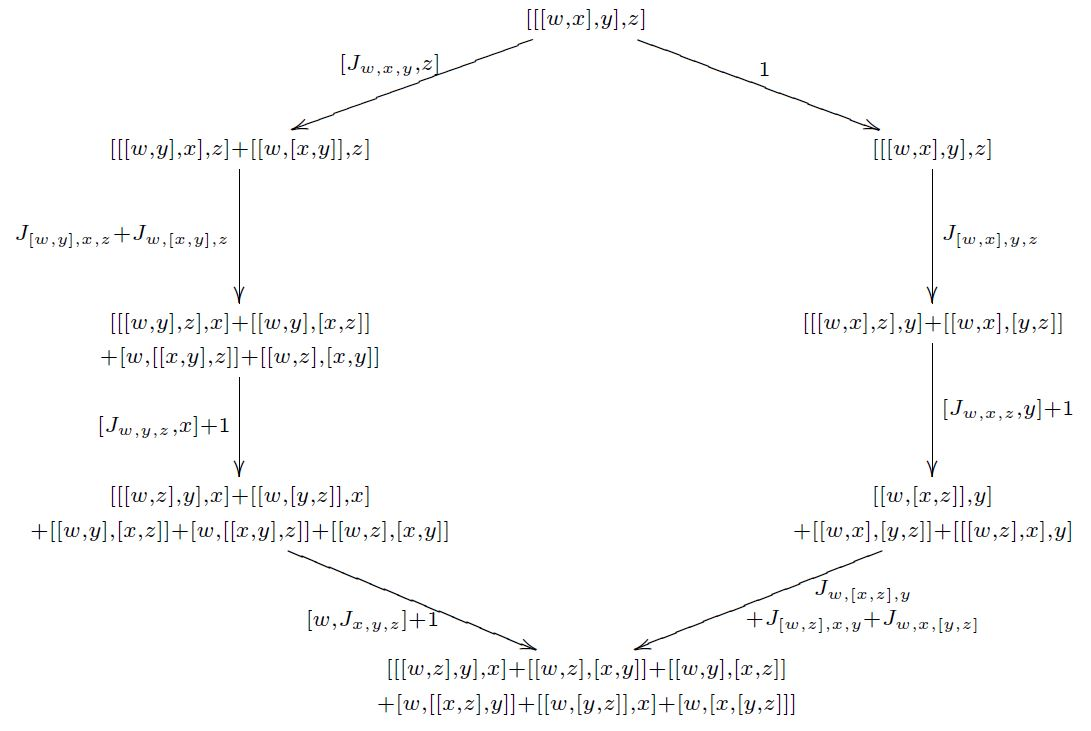(graphics grabbed from Baez-Crans 04, p. 19)

If the chain complex is concentrated in degrees 0 and 1 then this is all the data there is. This is a Lie 2-algebra (Baez-Crans 04)

In general there is a second-order homotopy filling this diagram, which in turn satisfies its own coherence condition up to a third-order homotopy, and so on.

$\,$

$\,$

The following example is trivial but important for the theory.

$\,$

Example. For $p \in \mathbb{N}$, there is a unique higher Lie algebra structure on the chain complex

$B^p \mathbb{R} \;\coloneqq\; \left[ \cdots \stackrel{0}{\to} \underset{\text{in degree} \atop {p}}{\underbrace{\mathbb{R}}} \stackrel{0}{\to} \cdots \stackrel{0}{\to} 0 \right]$

namely the one with vanishing bracket and vanishing higher homotopies. This is the line Lie (p+1)-algebra.

$\,$

What are higher Lie algebras good for?

1. they are equivalent to “formal moduli problems

controlling deformation theory (Hinich 97, Pridham 07, Lurie 1-, see Doubek-Markl-Zima 07)

2. they control closed string field theory (Zwiebach 93)

$\,$

$\,$

Higher extensions

$\,$

Higher Lie algebras turn out to be the answer to the question:

What do higher Lie algebra cocycles classify?

$\,$

A (p+2)-cocycle on a Lie algebra $(V,[-,-])$ is a function

$\mu_{p+2} \;\colon\; \underset{p+2\;\text{copies}}{\underbrace{V \times \cdots \times V}} \longrightarrow \mathbb{R}$

such that

1. multilinearity holds,

2. skew-symmetry holds,

3. cocycle condition: for all $(p+3)$-tuples $(x_0, \cdots, x_{p+2})$

$\underset{\sigma\in permutations}{\sum} (-1)^{sgn(\sigma)} \, \mu_{p+2}([x_{\sigma_0},x_{\sigma_1}], x_{\sigma_2}, \cdots, x_{\sigma_{p+2}}) = 0 \,.$

$\,$

Classical fact. Given a 2-cocycle $\mu_2$ it corresponds to a central extension Lie algebra extension, namely a Lie algebra structure on

$V \oplus \mathbb{R}$

given by

$[\, (x_1,c_1),\,(x_2,c_2) \,] \; \coloneqq \; (\, [x_1,x_2], \, \mu_2(x_1,x_2) \,) \,.$

For this bracket the Jacobi identity is equivalently the cocycle condition on $\mu_2$.

$\,$

Fact: For $p \in \mathbb{N}$ then a $(p+2)$-cocycle $\mu_{p+2}$ still defines an extension, but this is now a higher Lie algebra structure on the chain complex

$\left[ \cdots \overset{0}{\to} \underset{{in\, degree} \atop p}{\underbrace{\mathbb{R}}} \overset{0}{\to} \cdots \overset{0}{\to} V \right]$

whose Jacobiator and all its higher analogs are zero, except for the one of arity $(p+2)$, which is given by $\mu_{p+2}$.

$\,$

Example. Every semisimple Lie algebra $\mathfrak{g}$ carries a Killing form pairing

$\langle -,- \rangle \;\colon\; \mathfrak{g} \times \mathfrak{g} \longrightarrow \mathfrak{g}$

and the resulting trilinear map

$\mu_3 \coloneqq \langle -, [-,-] \rangle$

is a 3-cocycle.

This classifies a Lie 2-algebra extension of $\mathfrak{g}$,

called the string Lie 2-algebra

(The reason for this terminology we discuss below.)

$\,$ $\,$

Higher cocycles on higher Lie algebras

There are also $n$-cocycles on higher Lie algebras $\mathfrak{g}$.

In fact a $(p+2)$-cocycle on a higher Lie algebra $\mathfrak{g}$ is equivalently a homomorphism of higher Lie algebras of the form

$\mu_{p+2} \;\colon\; \mathfrak{g} \longrightarrow B^{p+1}\mathbb{R}$

and the higher Lie algebra extension $\widehat{\mathfrak{g}}$ that this classifies is equivalently the homotopy fiber

$\array{ \widehat{\mathfrak{g}} \\ {}^{\mathllap{hofib(\mu_{p+2})}}\downarrow \\ \mathfrak{g} &\stackrel{\mu_{p+2}}{\longrightarrow}& B^{p+1}\mathbb{R} }$

$\,$

This implies that from any higher Lie algebra $\mathfrak{g}$ there emanates a “bouquet” of consecutive higher extensions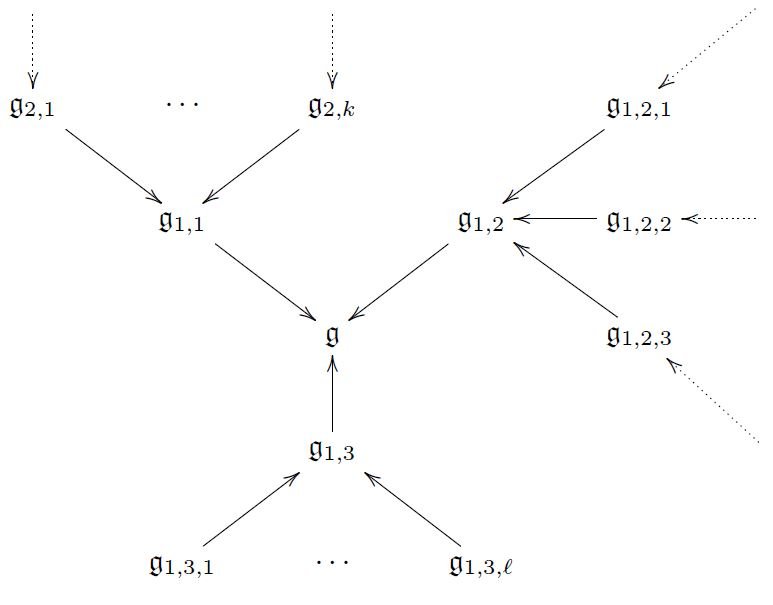$\,$

Fact. Such a higher homotopy bouquet controls the classification of super p-branes in string theory/M-theory (Fiorenza-Sati-Schreiber 13, Fiorenza-Sati-Schreiber 16).

$\,$

This shows that something deep relates higher structures with physics.

We come back to this at the end.

First we now explain how higher structures appear in physics.

$\,$

$\,$

## A higher structure at the heart of physics

$\,$

Classical physics is governed by the “principle of least action

(extremal action, really)

$\,$

This is formalized in variational calculus as follows.

Let

so that

• a field configuration is a section $\phi \in \Gamma_\Sigma(E)$

$\array{ && E \\ &{}^{\mathllap{\phi}}\nearrow& \downarrow \\ \Sigma &=& \Sigma }$

Write

• $J^\infty E$ for the jet bundle of $E$.

This means that if

$\array{ (& x^i, & v^a & ) \\ & {spacetime \atop coordinates} & {field \atop coordinates} } \;\;\;\;\;\;\;\;\;\;\;\;\; \text{chart of} \; E$

is a local coordinate chart of $E$, then the corresponding coordinate chart of the jet bundle is

$\array{ (& x^i, & v^a, & v^a_i, v^a_{i j}, \cdots & ) \\ & {spacetime \atop coordinates} & {field \atop coordinates} & {{partial\; derivatives}\atop {of\;field\; coordinates}} } \;\;\;\;\;\;\;\;\;\;\;\;\; \text{chart of} \; J^\infty E$

$\,$

$\,$

A local Lagrangian field theory is defined by a local Lagrangian density:

a $(p+1)$-form $L$ on $\Sigma$ which is a function of the fields and some finite number of its partial derivatives

$L(\,\{\text{fields}\}, \{\text{partial derivatives of fields}\}, \cdots \,) \in \Omega^{p+1}(\Sigma) \,.$

This is a horizontal differential $(p+1)$-form on the jet bundle of $E$.

$L \in \Omega^{p+1}_H(E) \subset \Omega^{p+1}(J^\infty E) \,.$

Here the horizontal differential $d_H$ is the “total derivative” given in the above coordinate chart by

$d_H = d x^i \left( \frac{\partial}{\partial x^i} + v^a_i \frac{\partial}{\partial v^a} + v^a_{i j} \frac{\partial}{\partial v^a_j} + \cdots \right)$

This defines a chain complex called the horizontal de Rham complex

$\left[ \Omega^0_H(E) \overset{d_H}{\longrightarrow} \Omega^1_H(E) \overset{d_H}{\longrightarrow} \cdots \overset{d_H}{\longrightarrow} \Omega^{p+1}_H(E) \right]$

Define the vertical differential on $J^\infty E$ to be

$d_V \coloneqq d_{dR} - d_H \,.$

This induces a chain complex of chain complexes, a double complex called the variational bicomplex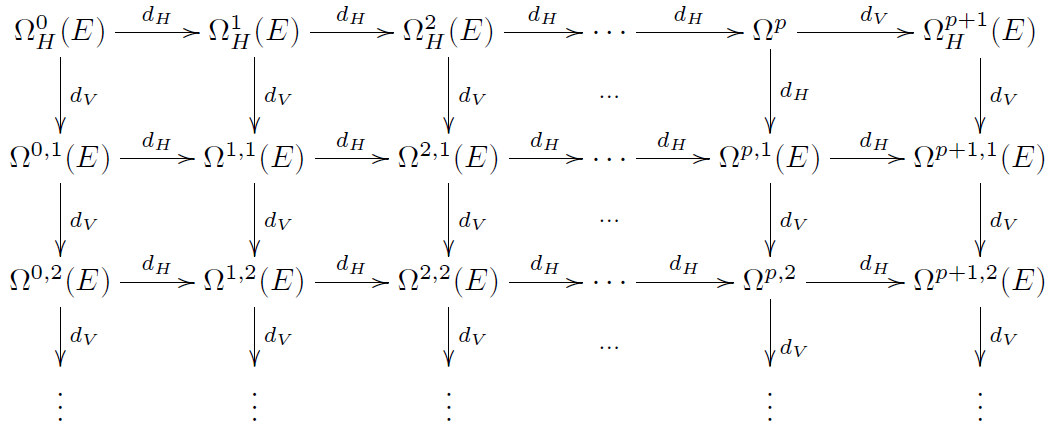$\,\,$

Say that the source forms are the wedge products of horizontal $(p+1)$-forms with vertical derivatives of functions of just the fields

$\Omega^{p+1,1}_S(E) \;\coloneqq\; \underset{top \atop horizontal}{\underbrace{\Omega^{p+1}_H(E)}} \wedge \underset{{vertical \; diff.} \atop {of \; field}}{\underbrace{d_V \Omega^0(E)}} \,.$

$\,$

Euler partial integration:

Source forms are a model for the top horizontal vertical 1-forms modulo horizontal divergences:

$\Omega^{p+1,1}_S(E) \simeq \Omega^{p+1,1}(E)/im(d_H) \,.$

Hence the full de Rham differential of any local Lagrangian density $L$ uniquely splits into a source-form and a horizontally exact term

$d L = \underset{source \atop form}{\underbrace{\delta_v L}} - \underset{horizontally \atop exact}{\underbrace{d_H \theta}}$

This $\delta_v$ is the variational derivative.

$\,$

The Euler-Lagrange equations of motion on field configurations $\phi$ is the partial differential equation

$(\delta_v L)(\phi) = 0$

hence

$\left( \frac{\partial}{\partial v^a} - \frac{\partial}{\partial x^i} \frac{\partial}{\partial v^a_i} + \frac{\partial^2}{\partial x^i \partial x^j} \frac{\partial}{\partial v^a_{i j}} - \cdots \right) L\left( \phi, \partial_i \phi, \partial_{i j}\phi, \cdots \right) \;\; = \;\; 0$

$\,$

$\,$

One checks immediately that

$\delta_v \circ d_H = 0 \,.$

Hence the horizontal de Rham complex continues to a longer chain complex

$\array{ \Omega^0_H(E) &\overset{d_H}{\longrightarrow}& \Omega^1_H(E) &\overset{d_H}{\longrightarrow}& \cdots &\overset{d_H}{\longrightarrow}& \Omega^p_H(E) &\overset{d_H}{\longrightarrow}& \Omega^{p+1}_H(E) \\ && && && && \downarrow^{\mathrlap{\delta_v}} \\ && && && && \Omega^{p+1,1}_S(E) }$

In fact it continues further:

$\array{ && && && & {\text{total} \atop \text{divergences}} & \\ \Omega^0_H(E) &\overset{d_H}{\longrightarrow}& \Omega^1_H(E) &\overset{d_H}{\longrightarrow}& \cdots &\overset{d_H}{\longrightarrow}& \Omega^p_H(E) &\overset{d_H}{\longrightarrow}& \Omega^{p+1}_H(E) \\ && && && && \downarrow^{\mathrlap{d_V}} & \text{Euler-Lagrange variational derivative} \\ && && && && \Omega^{n+1,1}(E)/im(d_H) \\ && && && && \downarrow^{\mathrlap{d_V}} & \text{Helmholtz operator} \\ && && && && \Omega^{p+1,2}(E)/im(d_H) \\ && && && && \downarrow^{\mathrlap{d_V}} \\ && && && && \vdots } \,.$

This is called the Euler-Lagrange complex.

$\,$

The Euler-Lagrange complex is locally exact. In other words, it participates in a variational version of the Poincaré lemma as above.

The Helmholtz operator on linear differential equations was found all the way back in (Helmholtz 1887).

$\,$

$\,$

This means for instance that

any given equation of motion $EL \in \Omega^{n+1,1}_S(E)$

is locally a “principle of extremal action

precisely if the Helmholtz operator annihilates it $d_V EL = 0$.

$\,$

$\,$

Hence the Euler-Lagrange complex is a higher structure at the heart of physics.

Question? What more do we gain by making it explicit this higher structure?

Surprising Answer: Many central examples of field theories may only be understood using this!

$\,$

This is due to

$\,$

## Three well-kept secrets

For many field theories of interest…Example $\;\;$
(1) …the Lagrangian density is
$\;\phantom{(3)}\;\;\;$ not globally defined.
charged particle in electromagnetic background field,
WZW model,
string in Kalb-Ramond field,
membrane in supergravity C-field,
D-brane in Kalb-Ramond field
(2) …the Lagrangian density is
$\;\phantom{(3)}\;\;\;$ a higher connection on a higher bundle
.
Wilson loop,
Wilson surface,
etc.,
(3) …the field bundle is itself a higher bundle.gauge theory such as Yang-Mills theory, Chern-Simons theory,
higher gauge theory such as AKSZ sigma-model
(Fiorenza-Rogers-Schreiber 11),
7d Chern-Simons theory of String 2-connection field
(Fiorenza-Sati-Schreiber 14)
$\;\phantom{(3)}\;$ …all three of these apply.electromagnetism with electron source fields,
RR-fields with D-brane source fields
(amplified in Freed 00)
super p-brane with tensor multiplet
such as
- D-branes with Chan-Paton gauge fields
- M5-brane with worldvolume gerbe
(Fiorenza-Sati-Schreiber 13)

$\,$

$\,$

We now explain these items in turn.

$\,$

### First secret: Locally variational theories

$\,$

Many local Lagrangian densities of interest are not actually globally defined.

These field theories are only locally variational

(in the terminology ofAnderson-Duchamp 80, Ferraris-Palese-Winterroth 11).

$\,$

This means that

1. there exists

1. an open cover of the field bundle by coordinate charts
$\{U_i \to E\}$
1. a collection of Lagrangian densities
$L_i \in \Omega^{p+1}_H(U_i)$

on each of the charts,

2. such that

• they have a globally well defined variational derivative

$EL \in \Omega^{p+1,1}_S(E)$

in that

$EL|_{U_i} \, = \, \delta_v L_i \,.$

$\,$

Example: charged particle

Let

• $\Sigma = \mathbb{R}$ (the “worldline”)

• $(X,g)$ a 4d spacetime

• $E \coloneqq \Sigma \times X$ the field bundle,

so that a field configuration is equivalently a smooth function

$\phi \colon \mathbb{R} \longrightarrow X$

i.e. a trajectory of a particle in $X$.

• $X$ carrying an electromagnetic field given by a differential 2-form

$\omega \in \Omega^2_{cl}(X)$

called the Faraday tensor, which in a local coordinate chart

$\mathbb{R}^{3,1} \hookrightarrow X$

defines

an electric field $\vec E = \left[ \array{E_1 \\ E_2 \\ E_3} \right]$ and magnetic field $\vec B = \left[ \array{B_1 \\ B_2 \\ B_3} \right]$

via

\begin{aligned} \omega & = E_1 \; d x^1 \wedge d x^0 + E_2 \; d x^2 \wedge d x^0 + E_3 \; d x^3 \wedge d x^0 \\ & + \;\; B_1 \; d x^2 \wedge d x^3 + B_2 \; d x^3 \wedge d x^1 + B_3 \; d x^1 \wedge d x^2 \end{aligned}

Then

1. there exists

1. an open cover $\{ U_i \to X\}$

2. a collection of 1-forms $A_i \in \Omega^1(U_i)$

2. such that

$\omega|_{U_i} = d_{dR} A_i$

and the locally defined Lagrangian density for the charged particle propagating in this background is

$L_i \coloneqq \left( \underset{(L_{kin})_i}{\underbrace{ g_{\mu \nu} \phi^\mu_t \phi^\nu_t }} + \underset{(L_{int})_i}{\underbrace{(A_i)_\mu \phi^\mu_t}} \right) d t \,.$

The Euler-Lagrange equations for this are the geodesic equations modified by the Lorentz force

$\array{ \delta_v L_i & = & \delta_v (L_{kin})_i &+& \delta_v (L_{int})_i \\ & = & \underset{\text{force of gravity}}\underbrace{ \phi^\mu_{t t} + \Gamma^\mu{}_{\alpha \beta} \phi^\alpha_t \phi^\beta_t } & +& \underset{\text{Lorentz force} \atop \text{of electromagnetic field}}{\underbrace{ F^\mu{}_\nu \phi^\nu_t }} = 0 }$

$\,$

$\,$

Hence there is an obvious question:

When may we find a globally defined Lagrangian for the charged particle?

$\,$

This is the kind of question that tools from homotopy theory naturally apply to.

after a closer look at the following…

$\,$

$\,$

### Second secret: Global action functionals

A collection of chartwise defined Lagrangians is not enough data to make sense of a globally defined exponentiated action functional

$\exp\left( \tfrac{i}{\hbar} \int_{\Sigma} L \right) \;\; \colon \Gamma_X(E) \longrightarrow \mathbb{R}/_{\hbar} \mathbb{Z} \simeq U(1) \,.$

Here Planck's constant $\hbar$ coordinatizes the circle group $U(1)$.

$0 \to \mathbb{Z} \stackrel{\hbar}{\longrightarrow} \mathbb{R} \stackrel{}{\longrightarrow} \underset{U(1)}{\underbrace{\mathbb{R}/_{\hbar} \mathbb{Z}}} \to 0$

$\,$

Instead, making sense of this requires to choose

• on each $(n+1)$-fold intersection of charts

• an order-$n$ higher homotopy

between the local Lagrangian densities: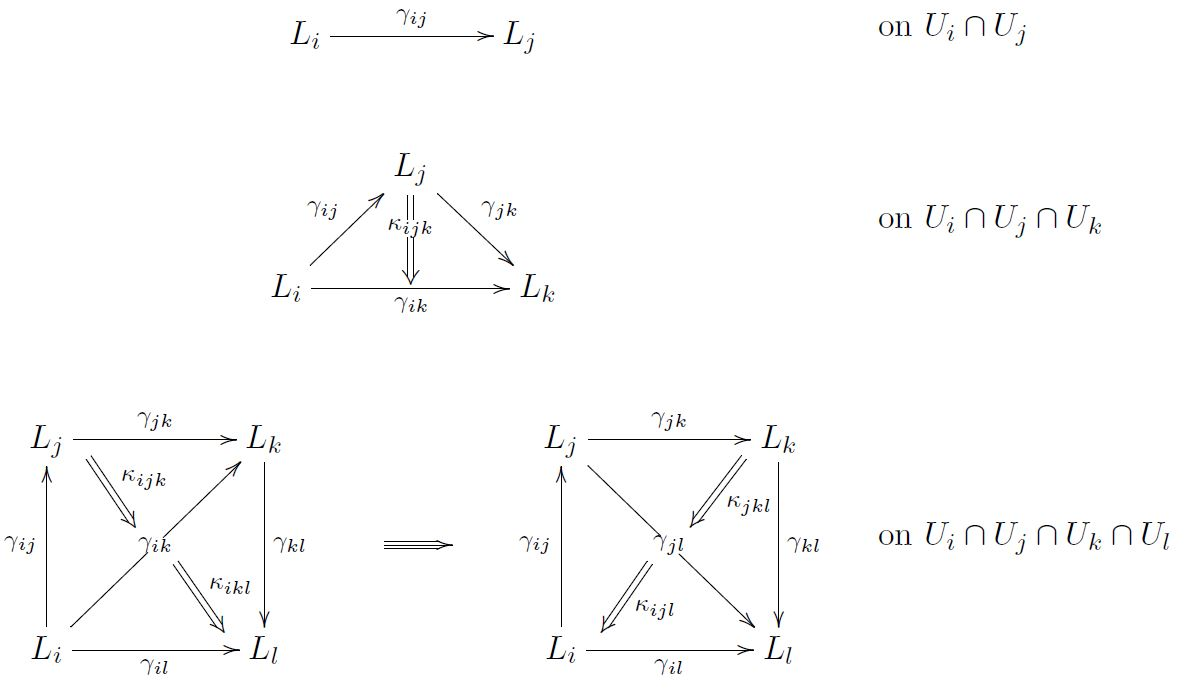and so on.

$\,$

Such a system of local Lagrangians with higher order homotopies between them is called

with coefficients in the horizontal Deligne complex:

$\left[ \mathbb{Z} \overset{\hbar}{\hookrightarrow} \Omega^0_H \overset{d_H}{\longrightarrow} \Omega^1_H \overset{d_H}{\longrightarrow} \cdots \overset{d_H}{\longrightarrow} \Omega^{p+1}_H \right] \,.$

$\,$

$\,$

Technical aside:

The statement here is that

$\,$

$\,$

The higher bundle underlying a local Lagrangian

$\,$

Underlying any such homotopically enhanced locally variational field theory $\mathbf{L}$ is a Cech cocycle representing an element

$c(\mathbf{L}) \;\in\; H^{p+2}(E,\mathbb{Z})$

in the integral cohomology of the field bundle

This is given by the evident chain homomorphism

$\array{ [ & \mathbb{Z} &\hookrightarrow& \Omega^0_H &\overset{d_H}{\longrightarrow}& \Omega^1_H &\overset{d_H}{\longrightarrow}& \cdots &\overset{d_H}{\longrightarrow}& \Omega^{p+1}_H & ] \\ & \downarrow^{\mathrm{id}} && \downarrow && \downarrow && && \downarrow \\ [ & \mathbb{Z} &\hookrightarrow& \Omega^0 &\overset{}{\longrightarrow}& 0 &\overset{}{\longrightarrow}& \cdots &\overset{}{\longrightarrow}& 0 & ] } \,.$

Here the chain complex

$\mathbf{B}^{p+1}U(1) \;\coloneqq\; \array{ [ & \mathbb{Z} &\hookrightarrow& \Omega^0 &\overset{}{\longrightarrow}& 0 &\overset{}{\longrightarrow}& \cdots &\overset{}{\longrightarrow}& 0 & ] }$

is a Lie (p+1)-group.

A cocycle as above classifies a higher principal bundle as above with higher structure group $\mathbf{B}^p U(1)$.

$\,$

Fact.

The obstruction to there being a globally defined Lagrangian density is the trivialization of this higher principal bundle, hence the vanishing

$c(\mathbf{L}) = 0 \;\;\;\;\in H^{p+2}(E,\mathbb{Z})$

of its characteristic class.

$\,$

Example

In the example of the charged particle above, this is the case precisely if, in physics speak:

This is the modern incarnation of the old Dirac charge quantization phenomenon (Dirac 31).

$\,$

$\,$

### Third secret: Higher field bundles

$\,$

For exposition see: Higher field bundles for gauge fields.

$\,$

$\,$

$\,$

## Higher Noether theorem

$\,$

Given a locally variational Lagrangian gerbe $\mathbf{L}$ as above, then a symmetry is a flow

$t \mapsto \exp(t v) \;\colon\; E \longrightarrow E$

along some vertical vector field

$v \in T_{vert} J^\infty E$

such that it preserves the Lagrangian density $\mathbf{L}$ in that the transformed Lagrangian density gerbe

$\exp(t v)^\ast \mathbf{L}$

coincides with $\mathbf{L}$.

In the spirit of homotopy theory, we need to choose a homotopy for each $t$

$exp(t v)^\ast \mathbf{L} \underoverset{\simeq}{\;\;\;\gamma(t)\;\;\;}{\longrightarrow} \mathbf{L} \,.$

An infinitesimal symmetry is obtained by differentiating this with respect to $t$. This yields

$\mathcal{L}_v L = d_H \gamma'$

where $\mathcal{L}$ denotes the Lie derivative.

By applying

1. Cartan's magic formula

2. Euler partial integration (above)

this becomes

$d_H \,(\, \underset{J}{\underbrace{\gamma' + \iota_v \theta}} \,) \;=\; \iota_v \delta_v \mathbf{L}$

Here $J$ is a conserved current

in that whenever the equations of motion hold

then its horizontal differential (total divergence) vanishes.

Hence symmetries of field theories induce conserved currents.

$\,$

But we could have made a different choice of homotopy

$(\exp(t v))^\ast L \underoverset{\simeq}{\;\;\;\gamma_2(t)\;\;\;}{\longrightarrow} L$

and we could have a higher order homotopy between them.

Higher Noether theorem There is an extension of the Lie algebra of symmetries by the higher Lie algebra of higher currents

$\left[ \array{ \Omega^0(E) \\ \downarrow^{d_H} \\ \cdots \\ \downarrow^{d_H} \\ \Omega^{p}_H(E)_{cl} } \right] \;\longrightarrow\; Cur(\mathbf{L}) \;\longrightarrow\; Sym(\mathbf{L})$

(Khavkine-Schreiber, based on Fiorenza-Rogers-Schreiber 14)

Passing to chain homology, this gives ordinary Lie algebra extension.

$0 \to H^{n-1}_{cl}(E) \longrightarrow H_0(Cur(\mathbf{L})) \longrightarrow Sym(\mathbf{L}) \to 0$

This is the cohomological version of the Noether theorem.

(Technically aside: The conserved currents here are the “conserved currents in characteristic form” in the terminology of Olver 86, after (4.29).)

$\,$

Example

Applied to Green-Schwarz sigma model for fundamental super p-branes this characterizes BPS states in supergravity. (Azcárraga-Gauntlett-Izquierdo-Townsend 89, Sati-Schreiber 15). This plays a major role in string theory.

$\,$

$\,$

To understand what is going on here, we now explain how these fundamental $p$-branes work:

## Example: Fundamental $p$-branes

$\,$

There are classes of examples of local variational local field theories for whose full analysis tools from higher structures are inevitable.

One such class is the generalization of the charged particle from above to higher dimensions,

$\,$

These have two kinds of applications

$\,$

$\,$

We close by discussing the archetypical case of a string propagating on a Lie group manifold.

$\,$

The fundamental string in a group manifold

Let $G$ be compact simply connected simple Lie group.

Such as

• the spin group $Spin(d)$ for $d \geq 3$

• the special unitary group $SU(n)$ for $n \geq 2$.

$\,$

We consider a “charged string” propagating on $G$, in direct analogy to the charged particle on $X$ from above.

$\,$

The higher analog of the Faraday tensor now is a differential 3-form.

Recall the Lie algebra 3-cocycle $\langle -,[-,-]\rangle$ from above above. By evaluating it on the Maurer-Cartan form

$\theta \in \Omega^1(G,\mathfrak{g})$

we obtain the closed left invariant differential form.

$\omega_3 = \langle \theta \wedge [\theta, \theta]\rangle$

Pulling this back to the jet bundle along

$J^\infty(\Sigma \times G) \longrightarrow G$

and projecting to the horizontal component yields the “Euler-Lagrane form for the higher Lorentz force”

$\omega_3|_H$

By the above obstruction theory we find:

there does not exist a globally defined Lagrangian density $L_{WZW}$ with $\delta_v L_{WZW} = (\mu_3)|_H$.

Instead: there exists a $U(1)$ bundle gerbe

$\mathbf{L}_{WZW} \in H(G, [\mathbb{Z} \to \Omega_H^0 \to \Omega_H^1 \to \Omega_H^2] )$

such that

$\delta_v \mathbf{L}_{WZW} = (\mu_3)|_H$

This is the jet bundle version (Khavkine-Schreiber) of a statement that goes back to (Gawedzki 87). For review see (Schweigert-Waldorf 07).

$\,$

Theorem The higher Noether current 2-group of this $\mathbf{L}_{WZW}$ sits in a homotopy fiber sequence of smooth infinity-groups

$\array{ \mathbf{B} U(1) &\longrightarrow& \mathbf{Cur}(\mathbf{L}_{WZW}) &\longrightarrow& G \\ && && \downarrow^{\mathrlap{\Omega \mathbf{c}_2}} \\ && && \mathbf{B}^2 U(1) } \,.$

$\,$

This identifies (Schreiber 13)

the higher Noether current group $Cur(\mathbf{L}_{WZW})$ of the string

whose Lie 2-algebra is the string Lie 2-algebra from above above

$\,$

$\,$

$\,$

Green-Schwarz anomaly cancellation

$\,$

Now let

$\array{ G &\hookrightarrow& P \\ && \downarrow \\ && X }$

be a $G$-principal bundle over some $X$.

We may ask for a Lagrangian that restricts on each fiber to the one above (a “parameterized WZW model”).

$\,$

Theorem (Distler-Sharpe 07, Schreiber 13) the obstruction to the parameterized WZW model on $P$ to exist is equivalently

1. a lift of $P$ to a principal 2-bundle (as above) for the string 2-group (from above)

2. vanishing of the canonical 4-class of $P$.

If $G = Spin(d) \times SU(n)^{op}$ then this 4-class is

$\tfrac{1}{2}p_1 - c_2$

The vanishing of this class is the Green-Schwarz anomaly cancellation in heterotic string theory.

$\,$

This is only the first step in a rich story.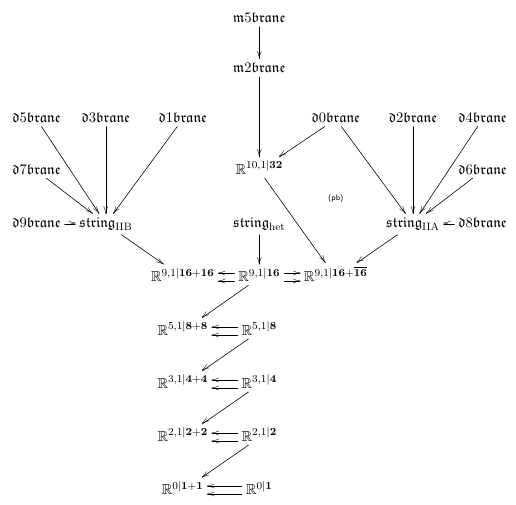$\,$

For more on this see the lecture notes

Pointers to original references are given in the above text. Here we just list surveys.

$\,$